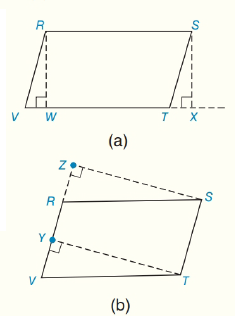Chapter 4.CT, Problem 7CTElementary Geometry For College St...

7th Edition
Alexander + 2 others
ISBN: 9781337614085

Solutions

Chapter
SectionElementary Geometry For College St...

7th Edition
Alexander + 2 others
ISBN: 9781337614085
Textbook Problem

Complete each statement:a) In ▭ R S T V , R W ¯ is the ________ from vertex R to base V T ¯ .b) If R W ¯ of figure (a) is congruent of T Y ¯ of figure (b), then ▭ R S T V must also bea(n)_______.To determine

(a)

To fill:

The given blanks.

Explanation

Given:

Definition:

An altitude of a parallelogram is a line segment from one vertex that is perpendicular to a non-adjacent side.

Approach:

From the figure, R is the vertex RW¯VT¯ and VT¯ is a non-adjacent side of R

To determine

(b)

To fill:

The given blanks.

Still sussing out bartleby?

Check out a sample textbook solution.

See a sample solution

The Solution to Your Study Problems

Bartleby provides explanations to thousands of textbook problems written by our experts, many with advanced degrees!

Get Started